# Kerala Syllabus 7th Standard Maths Solutions Chapter 11 Numbers and Algebra

You can Download Numbers and Algebra Questions and Answers, Activity, Notes, Kerala Syllabus 7th Standard Maths Solutions Chapter 11 help you to revise complete Syllabus and score more marks in your examinations.

## Kerala State Syllabus 7th Standard Maths Solutions Chapter 11 Numbers and Algebra

### Numbers and Algebra Text Book Questions and Answers

Odd and Even Textbook Page No. 146

Look at these sums:
1 + 2 = 3
2 + 3 = 5
3 + 4 = 7
All the sums are odd numbers, right?
Why is it that the sum of any two consecutive natural numbers is an odd number?
Let n be some natural number. Then the next natural number must be written n + 1.
What is their sum?
n + (n + 1) = 2n + 1
The number 2n + 1 divided by 2 gives quotient n and remainder 1.
So, whatever be the natural number n, the number 2n + 1 is odd.
Thus we can see that the sum of any two consecutive natural numbers is an odd number. Now look at these sums:
1 + 3 = 4
2 + 4 = 6
3 + 5 = 8
The sum of alternate natural numbers is an even number.
Can you explain this using algebra?
Let us consider the natural numbers to be n, 2n, 3n………
Since we are given alternate numbers we will consider the numbers n, 3n, 5n…..
n + 3n = 4n
Suppose when n = 1
1 + 3(1) = 4
Similarly, n = 2
2 + 3(2) = 6
When n = 3
3+ 3(3) = 12
Hence from the above Examples we can conclude “The sum of alternate natural numbers is an even number.” is true.

Number properties

We need algebra to show that certain properties are true for all numbers. For example, to prove that the sum of any two consecutive natural numbers is an odd number, we must first note that if a natural number is denoted by n, then the next natural number is n + 1 and that their sum is 2n + 1; also we must show that whatever be the natural number n, the number 2n + 1 is an odd number.

In many other sciences, if a certain fact is verified in a number of different contexts, then it is accepted as a general principle. This is not enough in mathematics. We must also show why it must be true in all cases. For numbers such reasoning is explained using algebra.

There are several instances where some facts are true for several numbers and then fail. For example if we divide 22 by 2, 23 by 3, 24 by 4 and so on, the remainder is not 3. Generally speaking, if we take any number less than 4700063497 as n, the number 2n divided by n will not leave remainder 3, but if we take 4700063497 as n we do get 3 as remainder.

Here a fact is true in more than four hundred seventy crore instances, but is not true in general.Three numbers Textbook Page No. 148

We have seen that the sum of two consecutive counting numbers is an odd number. What about the sum of three consecutive natural numbers?
1 + 2 + 3 = 6
2 + 3 + 4 = 9
3 + 4 + 5 = 12
All these are multiples of 3. Is this true, what ever number we start with?
If we write the first number as n, then the next numbers are n + 1 and n + 2.
Their sum is
n + (n + 1) + (n + 2) = 3n + 3
We can write
3n + 3 = 3 (n + 1)
So we can see that sum is a multiple of 3.
Here we can note another thing.

The sum is three times the middle number
Thus we get a more precise principle
The sum of any three consecutive counting numbers is three times the middle number
Is the sum of four consecutive counting numbers a multiple of four?
Let us consider the four consecutive numbers as n,n+1,n+2 and n+3. When they are added it gives the result as shown below
= n+n+1+n+2+n+3
= 4n+6
=2(2n+3)
Hence the result obtained is False since it is not a multiple of 4.

Another way

There is a another way to see that the sum of any three consecutive natural numbers is three times the middle number.
If the middle number is taken as n, then the first number is n – 1, and the last number is n + 1.
And their sum is
(n – 1) + n + (n + 1) = 3n
Here the convenience is that the sum of n – 1 and n + 1 can be easily seen to be 2n.

Now if we take any five consecutive a natural numbers and write the middle number as n, then the five numbers can be written as
n – 2, n – 1, n, n + 1, n + 2
To find the sum of all these we first note that
(n – 2) + (n + 2) = 2n
(n – 1) + ( n + 1) = 2n
Then the sum of all five can be easily found as
(n – 2) + (n – 1) + n + (n + 1) + (n + 2)
= 2n + 2n + n
= 5n
And this shows that the sum is five times the middle number.
What can you say about the sum of any seven consecutive natural numbers?
Let us consider the seven consecutive numbers as n-3,n-2,n-1,n,n+1,n+2, n+3. when they are added it gives the result as shown Below
= n-3+n-2+n-1+n+n+1+n+2+ n+3
= 7n-3-2-1+1+2+3
= 7n
Hence , the statement sum of any seven consecutive natural numbers is a multiple of 7 is True.General forms

The even numbers 2, 4, 6, 8, .. are all multiples of 2; that is, they are got by multiplying the natural numbers 1, 2, 3, 4, … by n. Thus for any natural number n, the number 2n is even; on other hand, any even number is of the form 2n.

If we subtract 1 from the even numbers 2, 4, 6, 8, …, we get the odd numbers. Generally speaking, the odd numbers are got by multiplying natural numbers by 2 and subtracting 1. Using algebra, the natural number n multiplied by 2 is written 2n and subtracting 1 gives 2n – 1. Thus for any natural number n, the number 2n – 1 is odd. On the other hand, any odd number can be written in the form 2n – 1.

For any natural number n, the number 2n + 1 is also odd. But if we take 1, 2, 3, … as n, we don’t get the number 1 from 2n + 1. To get all odd numbers from it, we should take 0, 1, 2, … as n.

Some other sums Textbook Page No. 151

If we add two even numbers, we get an even number; but if we add two odd numbers, still we get an even number. Why is this so?
Let’s use algebra. We can write any two even numbers as 2m, 2n. Their sum is
2m + 2n = 2 (m + n)
From this we see that the sum is also a multiple of 2; that is, an even number.

What about two odd numbers?
If we write them as 2m – 1, 2n – 1 then their sum is
(2m – 1) + (2n – 1) = 2m + 2n – 2
= 2(m + n – 1)
This again is a multiple of 2, or in other words an even number.
What if we add three even number instead of two? How about four even numbers?
What can you say about the sum of three odd numbers? What about four odd numbers?
Let 2m, 2n, 2o be the three even numbers, By adding the three numbers we get the result as
=2m +2n+ 2o
= 2(m+ n+ o)
Similarly if 2m, 2n, 2o, 2p be the three even numbers and the result obtained when added as
= 2m +2n+ 2o+2p
= 2(m+ n + o +p)
So, the sum of three even numbers and four Even numbers are multiples of 2.
Let 2m-1, 2n-1, 2o-1 be the three odd numbers, By adding the three numbers we get the result as
= 2m-1 +2n-1+ 2o-1
= 2m +2n+ 2o-3
Similarly if 2m-1,2n-1,2o-1,2p-1 be the four even numbers and the result obtained when added as
=2m-1+2n-1+2o-1+2p-1
=2m +2n+2o+2p-4
From the above result, the sum of three odd numbers and four odd numbers are not a multiple of 2.

Numbers and letters

When we use algebra to state general properties of numbers, we should also specify the type of numbers indicated by the letter.
For example, when we say that numbers of the form 2n – 1 are odd, we should also say that the n here indicates a natural number.
2n – 1 = (2 × 1$$\frac{1}{2}$$) – 1 = 2
Which is an even number.Algebraic Forms

It is easy to multiply any number by 10: just attach a zero at the end:
18 × 10 = 180
250 × 10 = 2500
But in algebra, we only write
10 × n = 10n
and not n0.
Similarly all numbers got by adding 1 to multiple of 10 end in 1. But we write their general algebraic form as 10n + 1 and not as n1.

Instead of saying, “numbers got by adding 1 to multiple of 10”, we can also say “numbers leaving remainder 1 on division by 10.” Such numbers leave remainder 1 on division by 5 also. To see this, note that
10n + 1 = (5 × 2n) + 1
If we write these numbers as n1, we cannot do such analysis.

Two-digit numbers Textbook Page No. 154

The number written as 35 is a short form for the number got by multiplying 3 by 10 and adding 5.
In general, the written form of a two-digit number actually denotes the number got by multiplying the first digit by 10 and adding second.
But in algebra, the result of multiplying the single digit number m by 10 and adding the single digit number n is written as 10m + n and not as mn.

But multiplying a single digit number n by 10 and adding the same number n can be written
10n + n = 11n
And from this we also see that all numbers got like this are multiples of 11.Three numbers again

Take any three consecutive natural numbers and add the first and last.
Is there any relation between this sum and the middle number?
Use algebra to prove that in any three consecutive natural numbers, the sum of the first and last is twice the middle one.

Is this true if we take three consecutive even numbers such as 2, 4, 6? How about consecutive odd numbers?
Next, is this true for any three consecutive multiples of 3, such as 3, 6, 9?
What about 1 added to multiples of 4 or any other number?
Prove all such conclusions using algebra.
(i) Given three consecutive numbers are 2, 4, 6
Middle Number = 4
Adding the first and last numbers we have 2+6 = 8
Thus, the sum of first and last numbers 2, 6 is equal to twice the middle number 4.
(ii) Given three consecutive multiples are 3, 6, 9
Middle Number = 6
Adding the first and last numbers we have 3+9 = 12
Thus, sum of first and last numbers 3, 9 is equal to twice the middle number 6.
(iii)Let us consider the multiples of 4 i.e. 4, 8, 12
Now adding 1 to all these multiples we have 4+1, 8+1, 12+1 = 5, 9, 13
Middle Number = 9
Now obtaining the sum of the first and last numbers = 5+13 = 18
Therefore, sum of first and last numbers 5, 13 is equal to twice the middle number 9.
Thus, we can conclude from the above numbers sum of first and last numbers is twice the middle number.

Number pyramid Textbook Page No. 146

Look at this picture: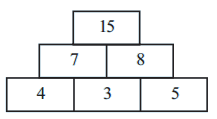Do you see any relation beween the numbers?
Pair of adjacent numbers in the bottom row are added to get the two numbers in the middle row; and these two numbers are added to get the top most number.
Let’s make such a pyramid starting with the numbers 1, 2, 3.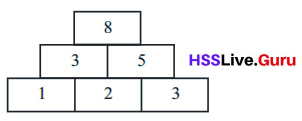What if we start with 2, 3, 4?Try with other sets of three consecutive natural numbers. Do the final numbers have anything in common?
What three consecutive numbers should we start with, to get 100 finally?
The three consecutive numbers to get 100 are 24, 25 and 26 are as shown Below
The numbers in the bottom row are 24, 25, and 26
To get the middle row numbers we will add the consecutive numbers i.e. 24+25 = 49, 25+26 = 51
Now, the top most row can be obtained by summing 49 and 51 i.e. 49+51 = 100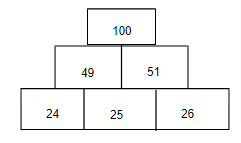Algebra aid

Whatever consecutive natural numbers we start with, our number pyramid ends in a multiple of 4, right?
Why does this happen?

Let’s take the first of these numbers as x.
Then the bottom row has x, x + 1, x + 2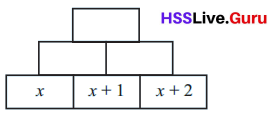What then are the numbers in the middle row?
x + (x + 1) = 2x + 1
(x + 1) + (x + 2) = 2x + 3So, what is the topmost number?
(2x + 1) + (2x + 3) = 4x + 4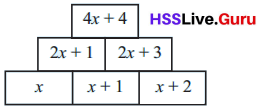Let’s write 4x + 4 in a slightly different way:
4x + 4 = 4(x + 1)
Thus, whatever three consecutive number we start with, we end up with four times the middle number (Did you
note this before?).
So to finish with 100, we should start with 24, 25, 26.
Now suppose we start with alternative numbers instead of consecutive numbers?
And three numbers skipping two each time?
Try these!

Can you complete these pyramids?Can you write the speciality of the last pyramid in ordinary language?
(i) Firstly, consider the numbers in the bottom row i.e. x-1, x, x+1
To obtain the middle row we will add x-1+x = 2x-1 and x+x+1 = 2x+1
Now the top most row is the summation of middle row i.e. 2x-1+2x+1 = 4x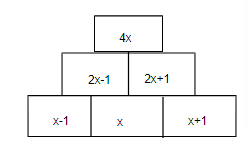(ii) Given pyramid has bottom row x-y, x, x+y
To get the middle row as per pyramid rule we have x-y+x = 2x-y, and  x+y+x = 2x+y
Now doing the sum of middle row numbers we will obtain the top most row i.e. 2x-y+2x+y = 4x-y+y =4xAs we can observe the middle number in bottom row is 4 times the number in topmost row of the pyramid.
Thus we can conclude the speciality of last pyramid is 4 times of the Last RowAnother pyramid Textbook Page No. 148

Here is a bigger pyramid: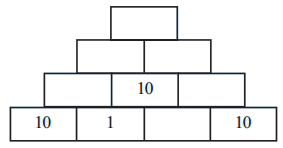Can you write the other numbers?
What number should be written in the remaining space of the bottom row?
1 added to that number should be 10.
Now can’t you write the remaining numbers?
Didn’t you get 50 at the top?
Given 1 added to the number is 10. Thus the number is 9
Filling the Space with 9 we have the Pyramid as such
To obtain the numbers in 2nd row we have 10+1 = 11, 1+9 = 10, 9+10 = 19
Summing the consecutive numbers in 2nd rows we have 11+10 = 21, 10+19 = 29
Now adding the numbers in 3rd row we get the topmost row number i.e.  21+29 = 50Next fill up this pyramid: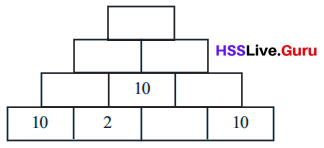The topmost number is still 50, right?
Given 2 added to the number is 10. Thus the number is 8
Filling the Space with 8 we have the Pyramid as such
To obtain the numbers in 2nd row we have 10+2 = 12, 2+8 = 10, 8+10 = 18
Summing the consecutive numbers in 2nd rows we have 12+10 = 22, 10+18 = 28
Now adding the numbers in 3rd row we get the topmost row number i.e.  22+28 = 50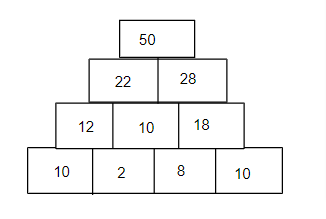Try with other numbers in the place of 2. Why do we always end up with 50?
Let us use 5 instead of 2 and obtain the numbers in each row
2nd row elements can be found as 10+5 = 15, 5+5 = 10, 5+10 = 15
3rd row elements can be obtained as 15+10 = 25, 10+15 = 25
Now the topmost row is 25+25 = 50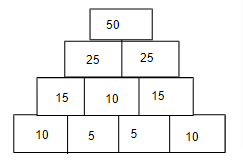Let’s use algebra and take the second number in the bottom row as x.
Then what should be the next number?Now can’t you fill up the next two row up?
Didn’t you get 20 + x, 30 – x in the third row?
So the top most number is
(20 + x) + (30 – x) = 50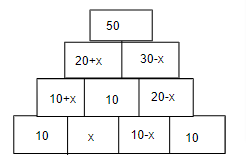Now let’s start with 9’s in the place of 10’s.
Take this,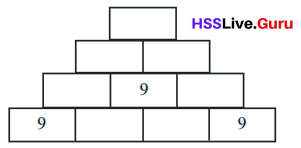Take any number less than 9 as the second number in the bottom row and fill in the pyramid (why less than 9?)
Compare with your friends.
All get 45, right?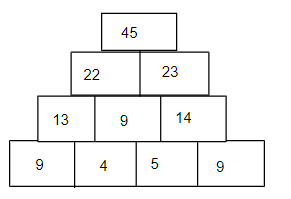Now if we start with 11 in the place of 9, can you say what number we would end up with, whatever number
(less than 11) we next take?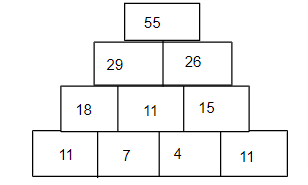Let us consider the number less than 11 is 7, 11-7 =4
So writing the Pyramid we have 3rd row as 18+11 = 29, 11+15 = 26
Now summing the 4th row 29+26 = 55
Why do we always get 5 times the beginning number?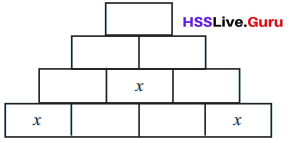Let’s write the beginning number as x.Let’s now take the next number as y:
What are the numbers in the next row up?
And in the next row after that ?
Didn’t you get 2x + y, 3x – y ? So the final number is
(2x + y) + (3x – y) = 5x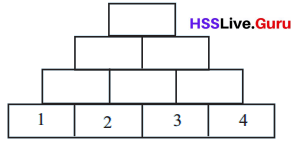• Fill in the pyramid below:
Given bottom row numbers are 1, 2, 3, 4
Numbers in the second row is 1+2 = 3, 2+3 = 5, 3+4 =7
Now numbers in the third row 3+5 = 8, 5+7 = 12
Numbers in the fourth row 8+12 = 20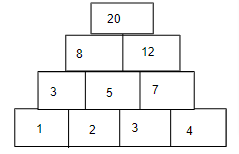(2+3)4 = 20

Try with other set of four consecutive natural numbers. What is the relation between the starting number and the final number? What is the relation between the middle two numbers in the bottom row and the top most number? Explain these relations using algebra.
Now let us consider the consecutive numbers are 4, 5, 6, 7
2nd row elements are 4+5 =9, 5+6 = 11, 6+7 = 13
3rd row elements 9+11 = 20, 11+13 = 24
4th row elements of the Pyramid are 20+24 = 44(5+6)×4 = 44

As we can see in both the cases sum of middle two numbers in the bottom row is 4 times the top most number.

• How about starting with five numbers?
Explain the relation between the middle number in the bottom row and number in the topmost row?• In the pyramids above, start with numbers skipping one at a time, two at a time and so on. Explain the various common relations you find using algebra.Given numbers are 1, 2, 3, 4, 5
2nd row elements in the Pyramid are 1+2 = 3, 2+3 =5, 3+4 = 7, 4+5 =9
3rd row elements in the Pyramid are 3+5 = 8, 5+7= 12, 7+9 = 16
4th row elements in the Pyramid are 8+12 = 20, 12+16=28
5th row element in the Pyramid is 20+28 = 48
Writing the Pyramid for these numbers we get as above.
Middle Number 3 in the Bottom Row of the Pyramid is 16 times the Topmost Number.Playing with 11

Look at these numbers:
12, 23, 34, …
Starting with 12, we add 11, then add 11 again and so on.
If we continue like this, would we get 100?
Let’s write and see:
12, 23, 34, 45, 56, 67, 78, 89, 100.
If we continue further, would we get 1000?
It is not easy to write them all.
Let’s have another look at the numbers.
12 is 1 more than 11.
23 is 1 more than22.
34 is 1 more than 33.
So all our numbers are got by adding 1 to multiples of 11.
In other words, they all leave remainder 1 on dividing by 11.
Now we can easily check whether 1000 is in this set.
The remainder on dividing 1000 by 11 is not 1. so 1000 is not among these numbers.
Next check whether 10000 is among them.

We first noted that all these numbers were got by starting with 12 and adding 11 repeatedly.
As seen now, this entire number pattern could be written as a single operation.
Multiply all natural numbers by 11 and add 1 to each.
How do we state this using algebra?
In 11n + 1, take n as 1, 2, 3, … in order.
(In algebra, natural numbers are usually denoted by letters like n, m, p, k; not a rule, just a convention).
Now instead of 12, let’s start with 21 and add 11 again and again:
21, 32, 43, …
Can we write this pattern also in algebra?
We can write them as
11 + 10, 22 + 10, 33 + 10, …
That is,
In 11n + 10, take n as 1, 2, 3, … in order.
If we continue this, which of the numbers 100, 1000, 10000 and 100000 do we get?
(What remainder do they leave on division by 11?)
Now let’s look at these patterns together: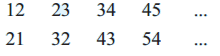What if we add the top and bottom numbers in order?
33 55 77 99 …
Why do we get only multiples of 11? Let’s check using algebra.
Any number in the first pattern can be written as 11 n + 1; the number in the same position in the second pattern is 11n + 10 (same n as the first)
What is their sum?
(11n + 1) + (11n + 10) = 22n + 11
= 11(2n + 1)

Now don’t you see why we get only multiples of 11?
Look at the sums again. Why do we get only odd multiple of 11?
Look at the algebraic form of the sum. When we take n as the natural numbers 1, 2, 3, … in it, what sort of numbers do we get as 2n + 1?

Here we see different general forms such as 11n + 1, 11n + 10, 2n + 1. Each indicates an operation. For example; 11n + 1 means multiply the number written as n by 11 and add 1.
Such general form indicating arithmetical operations are called algebraic expressions.
For example, all the numbers 12, 23, 34, … got by adding 11 repeatedly to 1 can be represented by the single algebraic expression 11n + 1.

• Find the algebraic expression for the numbers got by adding 10 repeatedly to 1
Given first number is 1.
When 10 is added to number 1 it gives the values as 11.
10+1 = 11
11+10 = 21
21+10 = 31
31+10 = 41
41+10= 51
.
.
.
.
Hence it is Expressed Algebraically as 10n+1 where n= 0, 1, 2, 3…

• Find the algebraic expression for the numbers got by adding 10 repeatedly to 9
Given first number is 9.
When 10 is added to number 9 it gives the values as 19
i.e. 9+10 = 19
19+10 = 29
29+10= 39
39+10 = 49
49+10=59
.
.
.
.
.
.
Hence it is Expressed Algebraically as 10n+9 where n = 0, 1, 2, 3….

• Add the numbers in the same position of the first two patterns. Why do we get only multiple of 10? Do we get all multiples of 10 like this?
We know the two series of numbers are 11, 21, 31, 41, 51, 61, ….. and 19, 29, 39, 49, 59…..
First number in the new series becomes 11+19 = 30
Second number in the new series becomes 21+29 = 50
Third number in the new series becomes 31+39 = 70
Adding the Algebraic Expressions we have (10n+1)+(10n+9) = 20n+10 =10(2n+1)
As we can see every term is a multiple of 10 but the series doesn’t cover all the multiples
Therefore, we don’t get all multiples of 10.

Two-digit numbers Textbook Page No. 153

We can write the multiples 10, 20, 30, .. of 10 in the com-mon form 10n. In it, we can take any natural number as n.
Suppose we need only the two-digit numbers among them. Then we need only take the numbers from 1 to 9. We write
10n (n = 1, 2, 3, 4, 5, 6, 7, 8, 9)
Or in a shorter from
10n (n = 1, 2, 3, …, 9)
Similarly the numbers 11,21, 31, … got by adding 1 to multiples of 10 can be shortened to the general form 10n + 1. In this we can take any natural number as n.
If we want only the two-digit number among these, we write
10n + 1 (n = 1, 2, 3, …, 9)

How do we write the general form of the numbers 12, 22, 32, … got by adding 2 to multiples of 10? And the two digit numbers among them?

Let’s take a look at all the two-digit numbers got so far:If we want all two digit numbers, what all algebraic ex¬pressions should we write?
What is the general form of the algebraic expressions, 10n, 10n + 1, 10n + 2, …, 10n + 9?
We add various numbers to be the expression 10n (the first number added is 0)
We can denote all these numbers added by a single letter, So we can write them as 10n + m; here we take the numbers from 0 to 9 as m.

In short, all two-digit numbers are of the form
10n + m (n = 1, 2, 3, ……, 9; m = 0, 1, 2, ……., 9)
For example if we take n = 5, m = 3 we get
10n + m = (10 × 5) + 3 = 53
What if we take n = 3, m = 5?

Thus in the general expression 10n + m for two digit numbers n is the first (place ten) digit and m is the second (place one) digit.
Now take any two-digit number say 25. Reversing the digits we get 52. Adding the two gives 77.
What about the sum of 36 and 63?
Do the digits repeat always?
How about 28 and 82?
Do all these sums have anything in common?
Why are they all multiples of 11?
To understand such common properties, algebra can be used.
Any two-digit number can be written in the form 10m + n.
Reversing the digits means interchanging their position and this gives 10n + m. Their sum is

(10m + n) + (10n + m) = (10m + m) + (10n + n)
= 11m + 11n
= 11(m + n)
Now reverse any two digit number and instead of adding, subtract smaller from the larger. Do it with other numbers.
Are all the differences, multiples of the same number?
Why does this happen?
(10m + n) – (10n + m) = 10m + n – 10n – m
= 9m – 9n
= 9(m – n)
Therefore, the differences is not the multiples of the same number.Now try these on your own:

• Take any two-digit number and add the digits. Subtract this sum from the number. Do this for several numbers and find a common property of all such differences.
Let us assume the two digit number as 23 and adding the digits we have 2+3 = 5
Subtracting this sum from the number we have taken 23-5 = 18
Now take one more two digit number 35 and adding the digits we have 3+5 =8
Subtracting this sum from the number we have taken 35-8 = 27
Let us assume one more number 47 and finding the sum we have 4+7 =11
Now subtracting the sum from number 47-11 = 36
Therefore, all the results are multiples of 9.

• If we subtract from any two digit number the sum of its digits, we get a multiple of 9. Explain why this is so using algebra.
Let us assume the two digit number to be ab i.e. 10a+b
Sum of its digits becomes a+b
Subtracting sum of its digits from two digit number we get 10a+b-(a+b)
= 9a+b-b
=9a
Hence Proved, on subtracting sum of its digits from any two digit number we get the multiple of 9.

• Write the general algebraic from of all three-digit numbers.
Let us consider the three digit number as xyz

• where x is the hundreds place
• y is the tens place
• z is the units place

The general algebraic form of all three digit numbers is 100x+10y+z

• If the first, second, third digits (in the hundreds, tens and ones place) of a three-digit number are taken as m, n, p, how do we write the number? How do we write the number got on reversing the digits?
Let us assume the three digit number as mnp

• where m is the hundreds place
• n is the tens place
• p is the units place

To write it as number we use the algebraic form 100m+10n+p

Now reversing the number we have pnm

• where p is the Hundreds place
• n is the tens place
• m is the units place

Now writing it as number we have 100p+10n+m

• If we reverse any three-digit number and subtract the smaller from the larger we get a multiple of 99. Explain this using algebra.
Let us consider the three digit number as xyz i.e. 100x+10y+z……(1)
Reversing the three digit number we have zyx i.e. 100z+10y+x…..(2)
Let us consider the 1st number to be larger and second number to be smaller we have the equation as such i.e. (100x+10y+z) – (100z+10y+x)
= 100x+10y+z-100z-10y-x
=99x-99z
=99(x-z)
Thus, when we reverse any three digit number and subtract the smaller from the larger we get a multiple of 99.

• From any three – digit number if we subtract the sum of its digits we get a multiple of 9. Explain this using algebra.